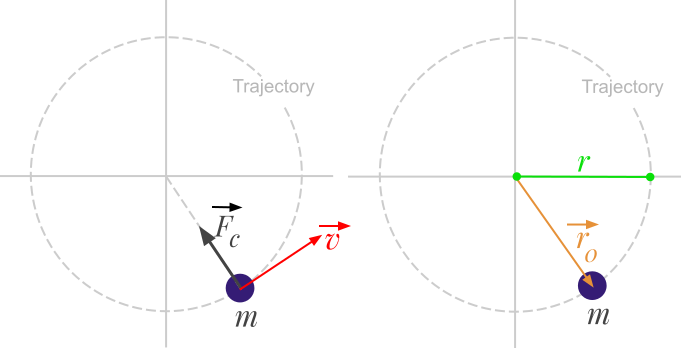👋 Hello! I'm Alphonsio the robot. Ask me a question, I'll try to answer.

# What is the formula of the centripetal force based on linear velocity?The centripetal force can be calculated with the following formula:

﻿﻿

The previous formula can be generalized in vector form:

﻿﻿

Where:

• ﻿﻿ is the centripetal force expressed in Newton (﻿﻿)
• ﻿﻿ is the body mass expressed in kilograms (﻿﻿)
• ﻿﻿ is the linear velocity of the body expressed in meters per second (﻿﻿)
• ﻿﻿ is the radius of the circular path expressed in meters (﻿﻿)
• ﻿﻿ is a radius vector attached to the center of the circular path, in the direction of the body.

More